# Texas Go Math Grade 5 Lesson 4.2 Answer Key Divide Decimals by Whole Numbers

Refer to our Texas Go Math Grade 5 Answer Key Pdf to score good marks in the exams. Test yourself by practicing the problems from Texas Go Math Grade 5 Lesson 4.2 Answer Key Divide Decimals by Whole Numbers.

## Texas Go Math Grade 5 Lesson 4.2 Answer Key Divide Decimals by Whole Numbers

Investigate

Materials; decimal models; color pencils

Angela has enough wood to make a picture frame with a perimeter of 2.4 meters. She wants the frame to be a square. What will be the length of each side of the frame?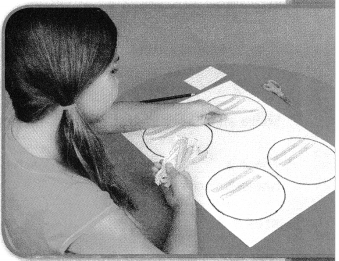A. Shade decimal models to show 2.4.

B. You need to share your model among __________ equal groups.

C. Since 2 wholes cannot be shared among 4 groups without regrouping, cut your model apart to show the tenths.
There are ______ tenths in 2.4.
Share the tenths equally among the 4 groups.
There are ______ ones and ______ tenths in each group.
Write a decimal for the amount in each group.

D. Use your model to complete the number sentence.
2.4 ÷ 4 = ___________
So, the length of each side of the frame will be ___________ meter.
A. Shade decimal models to show 2.4.
B. You need to share your model among  4 equal groups.
C. Since 2 wholes cannot be shared among 4 groups without regrouping, cut your model apart to show the tenths.
There are 24  tenths in 2.4.
Share the tenths equally among the 4 groups.
There are 0 ones and 6 tenths in each group.
Write a decimal for the amount in each group.
D. Use your model to complete the number sentence.
2.4 ÷ 4 =0.6
So, the length of each side of the frame will be 0.6 meter.
Explanation:
This is done by base 10 blocks model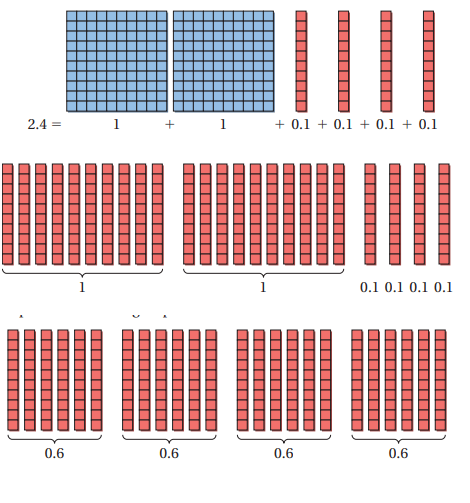Replace the ones blocks with tenths blocks. You have a total of 24 tenths blocks
Group the blocks into groups of 0.6 each.
There are four groups of 0.6. So, 2.4 ÷ 4 = 0.6

Draw Conclusions

Question 1.
You can also use area models to represent division. Shade the area model below and circle groups to show 2.4 ÷ 4.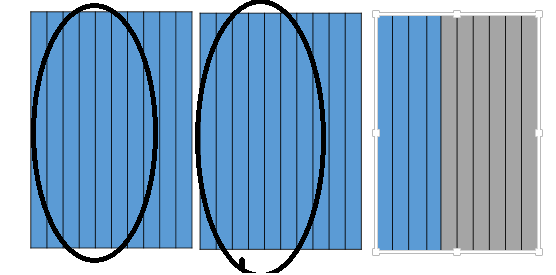Explanation:
Shaded the area model below and circle groups to show 2.4 ÷ 4.

Math Talk
Mathematical Processes

What other manipulatives or models could you use to solve this problem?
Explanation:
You can also use base 10 blocks method to model division of a decimal by whole number

Make Connections

You can also use base-ten blocks to model division of a decimal by a whole number.

Materials; base-ten blocks

Kyle has a roll of ribbon 3.21 yards long. He cuts the ribbon into 3 equal lengths. How long is each piece of ribbon?

Divide. 3.21 ÷ 3

STEP 1:
Use base-ten blocks to show 3.21.
Remember that a flat represents one, a long represents one tenth, and a small cube represents one hundredth.
There are __________ one(s), ________ tenth(s), and _________ hundredth(s).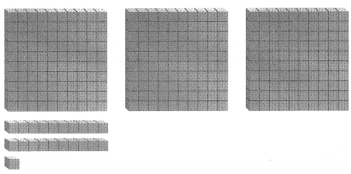STEP 2:
Share the ones.
Share an equal number of ones among 3 groups.
There is __________ one(s) shared in each group and _________ one(s) left over.

STEP 3:
Share the tenths.
Two tenths cannot be shared among 3 groups without regrouping. Regroup the tenths by replacing them with hundredths.
There are __________ tenth(s) shared in each group and __________ tenth(s) left over.
There are now __________ hundredth(s).STEP 4:
Share the hundredths.
Share the 21 hundredths equally among the 3 groups.
There are _________ hundredth(s) shared in each group and _________ hundredth(s) left over.
So, each piece of ribbon is __________ yards long.

STEP 1:
Remember that a flat represents one, a long represents one tenth, and a small cube represents one hundredth.
There are 3  one(s), 2  tenth(s), and 1 hundredth(s).STEP 2:
Share the ones.
Share an equal number of ones among 3 groups.
There is 3 one(s) shared in each group and 0 one(s) left over.

STEP 3:
Share the tenths.
Two tenths cannot be shared among 3 groups without regrouping. Regroup the tenths by replacing them with hundredths.
There are 0  tenth(s) shared in each group and 0 tenth(s) left over.
There are now 21 hundredth(s).STEP 4:
Share the hundredths.
Share the 21 hundredths equally among the 3 groups.
There are 21 hundredth(s) shared in each group and 0 hundredth(s) left over.
So, each piece of ribbon is 1.07 yards long.

Math Talk
Mathematical Processes

Explanation:
The sharing is to done by division.

Share and Show

Use the model to complete the number sentence.

Question 1.
1.6 ÷ 4 = ______________Explanation:
1.6 is divided into 4 groups equally
There is 1 one(s) cannot be shared in each group and 1 one(s) left over.
so, 16 tenths are divided in to 4 groups.
each group has 0.4

Question 2.
3.42 ÷ 3 = ____________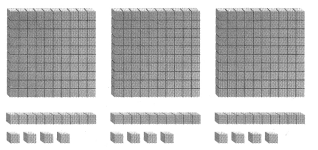Explanation:
This is done by base 10 blocks method
There are 3  one(s), 4  tenth(s), and 2 hundredth(s).
Shared an equal number of ones among 3 groups.
There is 3 one(s) shared in each group and 0 one(s) left over.
There are 4  tenth(s) shared in each group and 1 tenth(s) left over.
1 tenths cannot be regrouped so, done to hundredths
Share the 12 hundredths equally among the 3 groups.
There are 12 hundredth(s) shared in each group and 0 hundredth(s) left over.
Problem Solving

Question 3.
H.O.T. What’s the Error? Aida is making banners from a roll of paper that is 4.05 meters long. She will cut the paper into 3 equal lengths. She uses base-ten blocks to model how long each piece will be. Describe Aida’s error.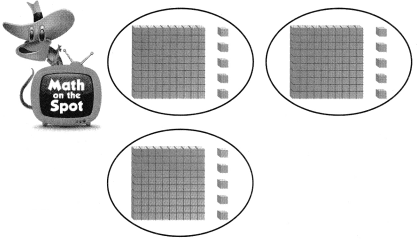Explanation:
She did not done tenths
There are 4  one(s), 0  tenth(s), and 5 hundredth(s).
Shared an equal number of ones among 3 groups.
There is 3 one(s) shared in each group and 1 one(s) left over.
There are 10  tenth(s) can be shared in each group and 1 tenth(s) left over.
1 tenths cannot be regrouped so, done to hundredths
Share the 15 hundredths equally among the 3 groups.
There are 15 hundredth(s) shared in each group and 0 hundredth(s) left over. 3 tenths is missing

Question 4.
Multi-Step Sam can ride his bike 4.5 kilometers in 9 minutes, and Amanda can ride her bike 3.6 kilometers in 6 minutes. Which rider might go farther in 1 minute?
Explanation:
Sam can ride his bike 4.5 kilometers in 9 minutes is 0.5
Amanda can ride her bike 3.6 kilometers in 6 minutes. is 0.6
so, 0.5 is greater than 0.6
Sam can ride faster in one minute

Question 5.
H.O.T. Explain how you can use inverse operations to find 1.8 ÷ 3.
Explanation:
To find the inverse operation we have to find multiplication
So, inverse operation for the equation is ____÷ 3 = 0.6

Question 6.
Multi-Step Yesterday, a bamboo plant was 12.62 yards tall. Today, the bamboo had grown by 0.34 yard. Bryson chopped the bamboo into 6 equal pieces. How long was each piece?
(A) 21.6 yd
(B) 0.216 yd
(C) 2.16 yd
(D) 216 yd
Explanation:
Total length of bamboo tree is 12.63+0.34=12.94,
Bryson chopped the bamboo tree into 6 equal parts
12.94÷6= 2.16 each piece was 2.16 long

Question 7.
Representations Terrance used base-ten blocks to help him divide a decimal by 4. His model is shown. What division problem did he model?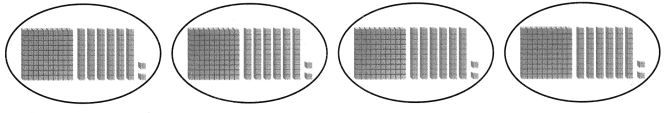(A) 1.26 ÷ 4
(B) 1.62 ÷ 4
(C) 6.48 ÷ 4
(D) 6.84 ÷ 4
Explanation:
There are 6 one(s), 4 tenth(s), and 8 hundredth(s).
Shared an equal number of ones among 4 groups.
There is 4 one(s) shared in each group and 2 one(s) left over.
There are  24 tenth(s) share in each group and 0 tenth(s) left over
There are 8 hundredth(s) shared in each group and 0 hundredth(s) left over.

Question 8.
Multi-Step Marvyn worked for 3 days. He earned $87.20 each day. He uses his earnings to buy four chairs. If he has no money left over, what is the cost of each chair? (A)$65.40
(B $261.60 (C)$116.27
(D) $21.80 Answer: D Explanation: Marvyn worked for 3 days. He earned$87.20 each day.
He uses his earnings to buy four chairs. If he has no money left over,
The cost of each chair is $21.80$87.30÷4=$21.80 Texas Test Prep Question 9. A bag of oranges costs$7.65. Five friends want to share the bag. How much will each friend pay?
(A) $38.25 (B)$1.53
(C) $2.00 (D)$1.13
Explanation:
A bag of oranges costs $7.65. Five friends want to share the bag.$1.53 should each friend pay
$7.65 ÷ 5 =$1.53

### Texas Go Math Grade 5 Lesson 4.2 Homework and Practice Answer Key

Use the model to complete the number sentence.

Question 1.
1.5 ÷ 3 = _________Explanation:
There are 1 one(s), 5 tenth(s), and 0 hundredth(s).
Shared an equal number of ones among 3 groups.
There is 1 one(s) cannot be shared in each group and 1 one(s) left over.
There are 10  tenth(s) can be shared in each group and 1 tenth(s) left over.
1 tenths cannot be regrouped so, done to hundredths
Share the 15 hundredths equally among the 3 groups.
There are 15 hundredth(s) shared in each group and 0 hundredth(s) left over.

Question 2.
2.48 ÷ 4 = __________Explanation: There are 2 one(s), 4  tenth(s), and 8 hundredth(s).
Shared an equal number of ones among 4 groups.
There is 2 one(s) cannot be shared in each group and 2 one(s) left over.
There are 24  tenth(s) can be shared in each group and 0 tenth(s) left over.
Share the 8 hundredths equally among the 4 groups.
There are 8 hundredth(s) shared in each group and 0 hundredth(s) left over.

Question 3.
2.8 ÷ 4 = ____________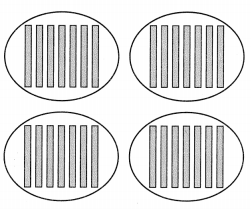Explanation: There are 2 one(s), 8 tenth(s), and 0 hundredth(s).
Shared an equal number of ones among 4 groups.
There is 2 one(s) cannot be  shared in each group and 2 one(s) left over.
There are 28  tenth(s) can be shared in each group and 0 tenth(s) left over.
Each group get 7 tenth parts equally

Question 4.
3.54 ÷ 3 = ____________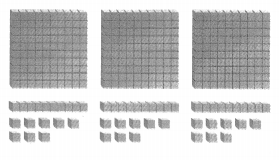Explanation:

Question 5.There are 3  one(s), 5  tenth(s), and 4 hundredth(s).
Shared an equal number of ones among 3 groups.
There is 3 one(s) shared in each group and 0 one(s) left over.
There are 5  tenth(s) can be shared in each group and 2 tenth(s) left over.
2 tenths cannot be regrouped so, done to hundredths
Share the 24 hundredths equally among the 3 groups.
There are 24 hundredth(s) shared in each group and 0 hundredth(s) left over.

Explain how you can use the whole number expression 248 ÷ 4 to check that your answer to Exercise 2 is reasonable.

Explanation: There are 2 one(s), 4  tenth(s), and 8 hundredth(s).
Shared an equal number of ones among 4 groups.
There is 2 one(s) cannot be shared in each group and 2 one(s) left over.
There are 24  tenth(s) can be shared in each group and 0 tenth(s) left over.
Share the 8 hundredths equally among the 4 groups.
There are 8 hundredth(s) shared in each group and 0 hundredth(s) left over.
Used a whole number expression 248 ÷ 4= 62
This is reasonable

Problem Solving

Question 6.
Mrs. Tillman builds furniture. She saws a maple board that is 4.56 meters long into 4 equal lengths. She saws an oak board that is 3.69 meters long into 3 equal lengths. Which is longer, a piece of oak or a piece of maple? Explain.

Explanation:
Mrs. Tillman builds furniture. She saws a maple board that is 4.56 meters long into 4 equal lengths. That is 1.14
She saws an oak board that is 3.69 meters long into 3 equal lengths. That is 1.23
A piece of oak is longer

Question 7.
Drew has a video game with five different challenges. He sets the timer to play his game for 10.75 minutes. He spends the same amount of time playing each challenge. How long does Drew play the fifth challenge?
Explanation: Drew has a video game with five different challenges.
He sets the timer to play his game for 10.75 minutes.
He spends the same amount of time playing each challenge.
So, 10.75 ÷ 5= 2.15 he plays the fifth challenge for 2.15 minutes

Lesson Check

Question 8.
Karina places concrete blocks along one side of her garden. The length of the border is 3.6 meters. If she uses 9 concrete blocks, what is the length of 1 block?
(A) 0.3 meter
(B) 0.9 meter
(C) 0.04 meter
(D) 0.4 meter
Explanation:
Karina places concrete blocks along one side of her garden.
The length of the border is 3.6 meters. If she uses 9 concrete blocks,
The length of 1 block is 0.4 meters

Question 9.
Max used base-ten blocks to solve the division problem 12.15 ÷ 3. Which set of blocks shows the quotient for 12.15 ÷ 3?
(A) 4 ones, 5 tenths, 0 hundredths
(B) 4 ones, 0 tenths, 5 hundredths
(C) 0 ones, 4 tenths, 5 hundredths
(D) 5 ones, 0 tenths, 4 hundredths
Explanation:
4 ones, 0 tenths and 5 hundredths shows the quotient for 12.53 that is divided by 3

Question 10.
The base-ten blocks pictured below show Carter’s solution to a division expression.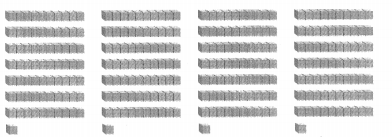Which expression did Carter solve?
(A) 2.84 ÷ 4
(B) 0.71 ÷ 4
(C) 2.48 ÷ 4
(D) 7.1 ÷ 4
Explanation:
There are 2 one(s), 8 tenth(s), and 4 hundredth(s).
Shared an equal number of ones among 4 groups.
There is 2 one(s) cannot be shared in each group and 2 one(s) left over.
There are 28  tenth(s) can be shared in each group and 0 tenth(s) left over. 4×7=28
Share the 4 hundredths equally among the 4 groups. 4×1=4
There are 4 hundredth(s) shared in each group and 0 hundredth(s) left over

Question 11.
Mr. Jefferson gives his two children $5.46 to spend at the garage sale. If they split the money evenly, how much will each child have to spend? (A)$1.82
(B) $1.23 (C)$2.23
(D) $2.73 Answer: D Explanation: Mr. Jefferson gives his two children$5.46 to spend at the garage sale.
If they split the money evenly,  Each child have to spend $2.73 Question 12. Multi-Step Mrs. Gonzales decorates the perimeter of her rectangular bulletin board with ribbon. She cuts a ribbon 3.72 yards long in half to decorate the top and the bottom. She cuts a ribbon 2.6 yards long in half to decorate the two sides. How much shorter is one side ribbon than the top ribbon? (A) 0.56 yard (B) 0.5 yard (C) 0.66 yard (D) 0.6 yard Answer: 1.86 and 1.3 = 0.56 (A) Explanation: Mrs. Gonzales decorates the perimeter of her rectangular bulletin board with ribbon. She cuts a ribbon 3.72 yards long in half to decorate the top and the bottom. That is 1.86 She cuts a ribbon 2.6 yards long in half to decorate the two sides. That is 1.3 1.86 – 1.3 = 0.56 yard shorter is one side ribbon than the top ribbon Question 13. Multi-Step Tamilca bought 6 fish at the pet store for a total of$7.26. If two of the fish together cost $4.10, and each of the other four fish had the same cost, how much was each remaining fish? (A)$1.21
(B) $2.05 (C)$0.79
(D) $3.16 Answer: C Explanation: Tamilca bought 6 fish at the pet store for a total of$7.26.
If two of the fish together cost $4.10, 7.26 – 4.10 = 3.16 and each of the other four fish had the same cost, 3.16÷ 4 =$0.79
The remaining fish cost each is \$0.79

Scroll to Top